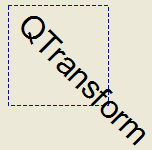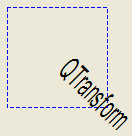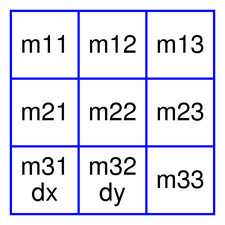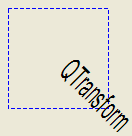# QTransform Class

The QTransform class specifies 2D transformations of a coordinate system. More...

 Header: #include CMake: find_package(Qt6 COMPONENTS Gui REQUIRED) target_link_libraries(mytarget PRIVATE Qt6::Gui) qmake: QT += gui

## Public Types

 enum TransformationType { TxNone, TxTranslate, TxScale, TxRotate, TxShear, TxProject }

## Public Functions

 QTransform(qreal m11, qreal m12, qreal m21, qreal m22, qreal dx, qreal dy) QTransform(qreal m11, qreal m12, qreal m13, qreal m21, qreal m22, qreal m23, qreal m31, qreal m32, qreal m33) QTransform() QTransform & operator=(const QTransform &matrix) qreal m11() const qreal m12() const qreal m13() const qreal m21() const qreal m22() const qreal m23() const qreal m31() const qreal m32() const qreal m33() const QTransform adjoint() const qreal determinant() const qreal dx() const qreal dy() const QTransform inverted(bool *invertible = nullptr) const bool isAffine() const bool isIdentity() const bool isInvertible() const bool isRotating() const bool isScaling() const bool isTranslating() const void map(qreal x, qreal y, qreal *tx, qreal *ty) const QPoint map(const QPoint &point) const QPointF map(const QPointF &p) const QLine map(const QLine &l) const QLineF map(const QLineF &line) const QPolygonF map(const QPolygonF &polygon) const QPolygon map(const QPolygon &polygon) const QRegion map(const QRegion ®ion) const QPainterPath map(const QPainterPath &path) const void map(int x, int y, int *tx, int *ty) const QRectF mapRect(const QRectF &rectangle) const QRect mapRect(const QRect &rectangle) const QPolygon mapToPolygon(const QRect &rectangle) const void reset() QTransform & rotate(qreal angle, Qt::Axis axis = Qt::ZAxis) QTransform & rotateRadians(qreal angle, Qt::Axis axis = Qt::ZAxis) QTransform & scale(qreal sx, qreal sy) void setMatrix(qreal m11, qreal m12, qreal m13, qreal m21, qreal m22, qreal m23, qreal m31, qreal m32, qreal m33) QTransform & shear(qreal sh, qreal sv) QTransform & translate(qreal dx, qreal dy) QTransform transposed() const QTransform::TransformationType type() const QVariant operator QVariant() const bool operator!=(const QTransform &matrix) const QTransform operator*(const QTransform &matrix) const QTransform & operator*=(const QTransform &matrix) QTransform & operator*=(qreal scalar) QTransform & operator+=(qreal scalar) QTransform & operator-=(qreal scalar) QTransform & operator/=(qreal scalar) bool operator==(const QTransform &matrix) const

## Static Public Members

 bool qFuzzyCompare(const QTransform &t1, const QTransform &t2) size_t qHash(const QTransform &key, size_t seed = 0) QPoint operator*(const QPoint &point, const QTransform &matrix) QPointF operator*(const QPointF &point, const QTransform &matrix) QLineF operator*(const QLineF &line, const QTransform &matrix) QLine operator*(const QLine &line, const QTransform &matrix) QPolygonF operator*(const QPolygonF &polygon, const QTransform &matrix) QPolygon operator*(const QPolygon &polygon, const QTransform &matrix) QRegion operator*(const QRegion ®ion, const QTransform &matrix) QPainterPath operator*(const QPainterPath &path, const QTransform &matrix) QDataStream & operator<<(QDataStream &stream, const QTransform &matrix) QDataStream & operator>>(QDataStream &stream, QTransform &matrix)

## Detailed Description

A transformation specifies how to translate, scale, shear, rotate or project the coordinate system, and is typically used when rendering graphics.

A QTransform object can be built using the setMatrix(), scale(), rotate(), translate() and shear() functions. Alternatively, it can be built by applying basic matrix operations. The matrix can also be defined when constructed, and it can be reset to the identity matrix (the default) using the reset() function.

The QTransform class supports mapping of graphic primitives: A given point, line, polygon, region, or painter path can be mapped to the coordinate system defined by this matrix using the map() function. In case of a rectangle, its coordinates can be transformed using the mapRect() function. A rectangle can also be transformed into a polygon (mapped to the coordinate system defined by this matrix), using the mapToPolygon() function.

QTransform provides the isIdentity() function which returns `true` if the matrix is the identity matrix, and the isInvertible() function which returns `true` if the matrix is non-singular (i.e. AB = BA = I). The inverted() function returns an inverted copy of this matrix if it is invertible (otherwise it returns the identity matrix), and adjoint() returns the matrix's classical adjoint. In addition, QTransform provides the determinant() function which returns the matrix's determinant.

Finally, the QTransform class supports matrix multiplication, addition and subtraction, and objects of the class can be streamed as well as compared.

### Rendering Graphics

When rendering graphics, the matrix defines the transformations but the actual transformation is performed by the drawing routines in QPainter.

By default, QPainter operates on the associated device's own coordinate system. The standard coordinate system of a QPaintDevice has its origin located at the top-left position. The x values increase to the right; y values increase downward. For a complete description, see the coordinate system documentation.

QPainter has functions to translate, scale, shear and rotate the coordinate system without using a QTransform. For example:```void SimpleTransformation::paintEvent(QPaintEvent *) { QPainter painter(this); painter.setPen(QPen(Qt::blue, 1, Qt::DashLine)); painter.drawRect(0, 0, 100, 100); painter.rotate(45); painter.setFont(QFont("Helvetica", 24)); painter.setPen(QPen(Qt::black, 1)); painter.drawText(20, 10, "QTransform"); }```

Although these functions are very convenient, it can be more efficient to build a QTransform and call QPainter::setTransform() if you want to perform more than a single transform operation. For example:```void CombinedTransformation::paintEvent(QPaintEvent *) { QPainter painter(this); painter.setPen(QPen(Qt::blue, 1, Qt::DashLine)); painter.drawRect(0, 0, 100, 100); QTransform transform; transform.translate(50, 50); transform.rotate(45); transform.scale(0.5, 1.0); painter.setTransform(transform); painter.setFont(QFont("Helvetica", 24)); painter.setPen(QPen(Qt::black, 1)); painter.drawText(20, 10, "QTransform"); }```

### Basic Matrix OperationsA QTransform object contains a 3 x 3 matrix. The `m31` (`dx`) and `m32` (`dy`) elements specify horizontal and vertical translation. The `m11` and `m22` elements specify horizontal and vertical scaling. The `m21` and `m12` elements specify horizontal and vertical shearing. And finally, the `m13` and `m23` elements specify horizontal and vertical projection, with `m33` as an additional projection factor.

QTransform transforms a point in the plane to another point using the following formulas:

```x' = m11*x + m21*y + dx
y' = m22*y + m12*x + dy
if (!isAffine()) {
w' = m13*x + m23*y + m33
x' /= w'
y' /= w'
}```

The point (x, y) is the original point, and (x', y') is the transformed point. (x', y') can be transformed back to (x, y) by performing the same operation on the inverted() matrix.

The various matrix elements can be set when constructing the matrix, or by using the setMatrix() function later on. They can also be manipulated using the translate(), rotate(), scale() and shear() convenience functions. The currently set values can be retrieved using the m11(), m12(), m13(), m21(), m22(), m23(), m31(), m32(), m33(), dx() and dy() functions.

Translation is the simplest transformation. Setting `dx` and `dy` will move the coordinate system `dx` units along the X axis and `dy` units along the Y axis. Scaling can be done by setting `m11` and `m22`. For example, setting `m11` to 2 and `m22` to 1.5 will double the height and increase the width by 50%. The identity matrix has `m11`, `m22`, and `m33` set to 1 (all others are set to 0) mapping a point to itself. Shearing is controlled by `m12` and `m21`. Setting these elements to values different from zero will twist the coordinate system. Rotation is achieved by setting both the shearing factors and the scaling factors. Perspective transformation is achieved by setting both the projection factors and the scaling factors.

#### Combining Transforms

Here's the combined transformations example using basic matrix operations:```void BasicOperations::paintEvent(QPaintEvent *) { const double a = qDegreesToRadians(45.0); double sina = sin(a); double cosa = cos(a); QTransform scale(0.5, 0, 0, 1.0, 0, 0); QTransform rotate(cosa, sina, -sina, cosa, 0, 0); QTransform translate(1, 0, 0, 1, 50.0, 50.0); QTransform transform = scale * rotate * translate; QPainter painter(this); painter.setPen(QPen(Qt::blue, 1, Qt::DashLine)); painter.drawRect(0, 0, 100, 100); painter.setTransform(transform); painter.setFont(QFont("Helvetica", 24)); painter.setPen(QPen(Qt::black, 1)); painter.drawText(20, 10, "QTransform"); }```

The combined transform first scales each operand, then rotates it, and finally translates it, just as in the order in which the product of its factors is written. This means the point to which the transforms are applied is implicitly multiplied on the left with the transform to its right.

#### Relation to Matrix Notation

The matrix notation in QTransform is the transpose of a commonly-taught convention which represents transforms and points as matrices and vectors. That convention multiplies its matrix on the left and column vector to the right. In other words, when several transforms are applied to a point, the right-most matrix acts directly on the vector first. Then the next matrix to the left acts on the result of the first operation - and so on. As a result, that convention multiplies the matrices that make up a composite transform in the reverse of the order in QTransform, as you can see in Combining Transforms. Transposing the matrices, and combining them to the right of a row vector that represents the point, lets the matrices of transforms appear, in their product, in the order in which we think of the transforms being applied to the point.

## Member Type Documentation

### enum QTransform::TransformationType

ConstantValue
`QTransform::TxNone``0x00`
`QTransform::TxTranslate``0x01`
`QTransform::TxScale``0x02`
`QTransform::TxRotate``0x04`
`QTransform::TxShear``0x08`
`QTransform::TxProject``0x10`

## Member Function Documentation

### QTransform::QTransform(qrealm11, qrealm12, qrealm21, qrealm22, qrealdx, qrealdy)

Constructs a matrix with the elements, m11, m12, m21, m22, dx and dy.

### QTransform::QTransform(qrealm11, qrealm12, qrealm13, qrealm21, qrealm22, qrealm23, qrealm31, qrealm32, qrealm33)

Constructs a matrix with the elements, m11, m12, m13, m21, m22, m23, m31, m32, m33.

### QTransform::QTransform()

Constructs an identity matrix.

All elements are set to zero except `m11` and `m22` (specifying the scale) and `m33` which are set to 1.

### QTransform &QTransform::operator=(const QTransform &matrix)

Assigns the given matrix's values to this matrix.

### qreal QTransform::m11() const

Returns the horizontal scaling factor.

### qreal QTransform::m12() const

Returns the vertical shearing factor.

### qreal QTransform::m13() const

Returns the horizontal projection factor.

### qreal QTransform::m21() const

Returns the horizontal shearing factor.

### qreal QTransform::m22() const

Returns the vertical scaling factor.

### qreal QTransform::m23() const

Returns the vertical projection factor.

### qreal QTransform::m31() const

Returns the horizontal translation factor.

### qreal QTransform::m32() const

Returns the vertical translation factor.

### qreal QTransform::m33() const

Returns the division factor.

Returns the adjoint of this matrix.

### qreal QTransform::determinant() const

Returns the matrix's determinant.

### qreal QTransform::dx() const

Returns the horizontal translation factor.

### qreal QTransform::dy() const

Returns the vertical translation factor.

### `[static] `QTransform QTransform::fromScale(qrealsx, qrealsy)

Creates a matrix which corresponds to a scaling of sx horizontally and sy vertically. This is the same as QTransform().scale(sx, sy) but slightly faster.

### `[static] `QTransform QTransform::fromTranslate(qrealdx, qrealdy)

Creates a matrix which corresponds to a translation of dx along the x axis and dy along the y axis. This is the same as QTransform().translate(dx, dy) but slightly faster.

### QTransform QTransform::inverted(bool *invertible = nullptr) const

Returns an inverted copy of this matrix.

If the matrix is singular (not invertible), the returned matrix is the identity matrix. If invertible is valid (i.e. not 0), its value is set to true if the matrix is invertible, otherwise it is set to false.

### bool QTransform::isAffine() const

Returns `true` if the matrix represent an affine transformation, otherwise returns `false`.

### bool QTransform::isIdentity() const

Returns `true` if the matrix is the identity matrix, otherwise returns `false`.

### bool QTransform::isInvertible() const

Returns `true` if the matrix is invertible, otherwise returns `false`.

### bool QTransform::isRotating() const

Returns `true` if the matrix represents some kind of a rotating transformation, otherwise returns `false`.

Note: A rotation transformation of 180 degrees and/or 360 degrees is treated as a scaling transformation.

### bool QTransform::isScaling() const

Returns `true` if the matrix represents a scaling transformation, otherwise returns `false`.

### bool QTransform::isTranslating() const

Returns `true` if the matrix represents a translating transformation, otherwise returns `false`.

### void QTransform::map(qrealx, qrealy, qreal *tx, qreal *ty) const

Maps the given coordinates x and y into the coordinate system defined by this matrix. The resulting values are put in *tx and *ty, respectively.

The coordinates are transformed using the following formulas:

```x' = m11*x + m21*y + dx
y' = m22*y + m12*x + dy
if (!isAffine()) {
w' = m13*x + m23*y + m33
x' /= w'
y' /= w'
}```

The point (x, y) is the original point, and (x', y') is the transformed point.

### QPoint QTransform::map(const QPoint &point) const

Creates and returns a QPoint object that is a copy of the given point, mapped into the coordinate system defined by this matrix. Note that the transformed coordinates are rounded to the nearest integer.

### QPointF QTransform::map(const QPointF &p) const

Creates and returns a QPointF object that is a copy of the given point, p, mapped into the coordinate system defined by this matrix.

### QLine QTransform::map(const QLine &l) const

Creates and returns a QLineF object that is a copy of the given line, l, mapped into the coordinate system defined by this matrix.

### QLineF QTransform::map(const QLineF &line) const

Creates and returns a QLine object that is a copy of the given line, mapped into the coordinate system defined by this matrix. Note that the transformed coordinates are rounded to the nearest integer.

### QPolygonF QTransform::map(const QPolygonF &polygon) const

Creates and returns a QPolygonF object that is a copy of the given polygon, mapped into the coordinate system defined by this matrix.

### QPolygon QTransform::map(const QPolygon &polygon) const

Creates and returns a QPolygon object that is a copy of the given polygon, mapped into the coordinate system defined by this matrix. Note that the transformed coordinates are rounded to the nearest integer.

### QRegion QTransform::map(const QRegion &region) const

Creates and returns a QRegion object that is a copy of the given region, mapped into the coordinate system defined by this matrix.

Calling this method can be rather expensive if rotations or shearing are used.

### QPainterPath QTransform::map(const QPainterPath &path) const

Creates and returns a QPainterPath object that is a copy of the given path, mapped into the coordinate system defined by this matrix.

### void QTransform::map(intx, inty, int *tx, int *ty) const

Maps the given coordinates x and y into the coordinate system defined by this matrix. The resulting values are put in *tx and *ty, respectively. Note that the transformed coordinates are rounded to the nearest integer.

### QRectF QTransform::mapRect(const QRectF &rectangle) const

Creates and returns a QRectF object that is a copy of the given rectangle, mapped into the coordinate system defined by this matrix.

The rectangle's coordinates are transformed using the following formulas:

```x' = m11*x + m21*y + dx
y' = m22*y + m12*x + dy
if (!isAffine()) {
w' = m13*x + m23*y + m33
x' /= w'
y' /= w'
}```

If rotation or shearing has been specified, this function returns the bounding rectangle. To retrieve the exact region the given rectangle maps to, use the mapToPolygon() function instead.

### QRect QTransform::mapRect(const QRect &rectangle) const

Creates and returns a QRect object that is a copy of the given rectangle, mapped into the coordinate system defined by this matrix. Note that the transformed coordinates are rounded to the nearest integer.

### QPolygon QTransform::mapToPolygon(const QRect &rectangle) const

Creates and returns a QPolygon representation of the given rectangle, mapped into the coordinate system defined by this matrix.

The rectangle's coordinates are transformed using the following formulas:

```x' = m11*x + m21*y + dx
y' = m22*y + m12*x + dy
if (!isAffine()) {
w' = m13*x + m23*y + m33
x' /= w'
y' /= w'
}```

Polygons and rectangles behave slightly differently when transformed (due to integer rounding), so `matrix.map(QPolygon(rectangle))` is not always the same as `matrix.mapToPolygon(rectangle)`.

### `[static] `bool QTransform::quadToQuad(const QPolygonF &one, const QPolygonF &two, QTransform &trans)

Creates a transformation matrix, trans, that maps a four-sided polygon, one, to another four-sided polygon, two. Returns `true` if the transformation is possible; otherwise returns false.

### `[static] `bool QTransform::quadToSquare(const QPolygonF &quad, QTransform &trans)

Creates a transformation matrix, trans, that maps a four-sided polygon, quad, to a unit square. Returns `true` if the transformation is constructed or false if such a transformation does not exist.

### void QTransform::reset()

Resets the matrix to an identity matrix, i.e. all elements are set to zero, except `m11` and `m22` (specifying the scale) and `m33` which are set to 1.

### QTransform &QTransform::rotate(qrealangle, Qt::Axisaxis = Qt::ZAxis)

Rotates the coordinate system counterclockwise by the given angle about the specified axis and returns a reference to the matrix.

Note that if you apply a QTransform to a point defined in widget coordinates, the direction of the rotation will be clockwise because the y-axis points downwards.

The angle is specified in degrees.

### QTransform &QTransform::rotateRadians(qrealangle, Qt::Axisaxis = Qt::ZAxis)

Rotates the coordinate system counterclockwise by the given angle about the specified axis and returns a reference to the matrix.

Note that if you apply a QTransform to a point defined in widget coordinates, the direction of the rotation will be clockwise because the y-axis points downwards.

The angle is specified in radians.

### QTransform &QTransform::scale(qrealsx, qrealsy)

Scales the coordinate system by sx horizontally and sy vertically, and returns a reference to the matrix.

### void QTransform::setMatrix(qrealm11, qrealm12, qrealm13, qrealm21, qrealm22, qrealm23, qrealm31, qrealm32, qrealm33)

Sets the matrix elements to the specified values, m11, m12, m13 m21, m22, m23 m31, m32 and m33. Note that this function replaces the previous values. QTransform provides the translate(), rotate(), scale() and shear() convenience functions to manipulate the various matrix elements based on the currently defined coordinate system.

### QTransform &QTransform::shear(qrealsh, qrealsv)

Shears the coordinate system by sh horizontally and sv vertically, and returns a reference to the matrix.

### `[static] `bool QTransform::squareToQuad(const QPolygonF &quad, QTransform &trans)

Creates a transformation matrix, trans, that maps a unit square to a four-sided polygon, quad. Returns `true` if the transformation is constructed or false if such a transformation does not exist.

### QTransform &QTransform::translate(qrealdx, qrealdy)

Moves the coordinate system dx along the x axis and dy along the y axis, and returns a reference to the matrix.

### QTransform QTransform::transposed() const

Returns the transpose of this matrix.

### QTransform::TransformationType QTransform::type() const

Returns the transformation type of this matrix.

The transformation type is the highest enumeration value capturing all of the matrix's transformations. For example, if the matrix both scales and shears, the type would be `TxShear`, because `TxShear` has a higher enumeration value than `TxScale`.

Knowing the transformation type of a matrix is useful for optimization: you can often handle specific types more optimally than handling the generic case.

### QVariant QTransform::operator QVariant() const

Returns the transform as a QVariant.

### bool QTransform::operator!=(const QTransform &matrix) const

Returns `true` if this matrix is not equal to the given matrix, otherwise returns `false`.

### QTransform QTransform::operator*(const QTransform &matrix) const

Returns the result of multiplying this matrix by the given matrix.

Note that matrix multiplication is not commutative, i.e. a*b != b*a.

### QTransform &QTransform::operator*=(const QTransform &matrix)

Returns the result of multiplying this matrix by the given matrix.

### QTransform &QTransform::operator*=(qrealscalar)

Returns the result of performing an element-wise multiplication of this matrix with the given scalar.

### QTransform &QTransform::operator+=(qrealscalar)

Returns the matrix obtained by adding the given scalar to each element of this matrix.

### QTransform &QTransform::operator-=(qrealscalar)

Returns the matrix obtained by subtracting the given scalar from each element of this matrix.

### QTransform &QTransform::operator/=(qrealscalar)

Returns the result of performing an element-wise division of this matrix by the given scalar.

### bool QTransform::operator==(const QTransform &matrix) const

Returns `true` if this matrix is equal to the given matrix, otherwise returns `false`.

## Related Non-Members

### boolqFuzzyCompare(const QTransform &t1, const QTransform &t2)

Returns `true` if t1 and t2 are equal, allowing for a small fuzziness factor for floating-point comparisons; false otherwise.

### `[since 5.6] `size_tqHash(const QTransform &key, size_tseed = 0)

Returns the hash value for key, using seed to seed the calculation.

This function was introduced in Qt 5.6.

### QPointoperator*(const QPoint &point, const QTransform &matrix)

This is the same as matrix.map(point).

### QPointFoperator*(const QPointF &point, const QTransform &matrix)

Same as matrix.map(point).

### QLineFoperator*(const QLineF &line, const QTransform &matrix)

This is the same as matrix.map(line).

### QLineoperator*(const QLine &line, const QTransform &matrix)

This is the same as matrix.map(line).

### QPolygonFoperator*(const QPolygonF &polygon, const QTransform &matrix)

This is the same as matrix.map(polygon).

### QPolygonoperator*(const QPolygon &polygon, const QTransform &matrix)

This is the same as matrix.map(polygon).

### QRegionoperator*(const QRegion &region, const QTransform &matrix)

This is the same as matrix.map(region).

### QPainterPathoperator*(const QPainterPath &path, const QTransform &matrix)

This is the same as matrix.map(path).

### QDataStream &operator<<(QDataStream &stream, const QTransform &matrix)

Writes the given matrix to the given stream and returns a reference to the stream.